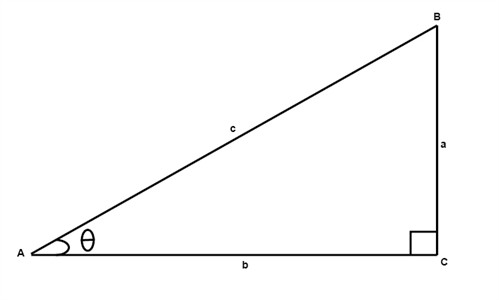# Trigonometric functions

Trigonometric functions are functions of an angle. They are used to relate the angles of a triangle to the lengths of the sides of a triangle.

If we have a right triangle with one angle $\theta$Then the following hold true

$sine\: \theta =\frac{a}{c}$

$cosine \:\theta =\frac{b}{c}$

$tangent \:\theta =\frac{a}{b}$

$cosecant \:\theta =\frac{c}{a}$

$secant \:\theta =\frac{c}{b}$

$cotangent \:\theta =\frac{b}{a}$

Example

If we are given one angle in a right triangle $\theta = 45^{\circ}$ and we know that the measure of the hypotenuse is 2, what are the measures of the two other legs?

Using the formulas above we get that:

$sine\; 45^{\circ}=\frac{a}{2}$

$cosine\; 45^{\circ}=\frac{b}{2}$

We use our calculators to determine sine $45^{\circ}$ and cosine $45^{\circ}$ :

$0.707=\frac{a}{2}$

$0.707=\frac{b}{2}$

Now we can see that the measures of $a$ and $b$ are equal and that the length is

$2 \cdot 0.707 = 1.414$

## Video lesson

If we are given one angle in a right triangle $\theta = 20^{\circ}$ and we know that the measure of the hypotenuse is 3, what are the measures of the two other legs?